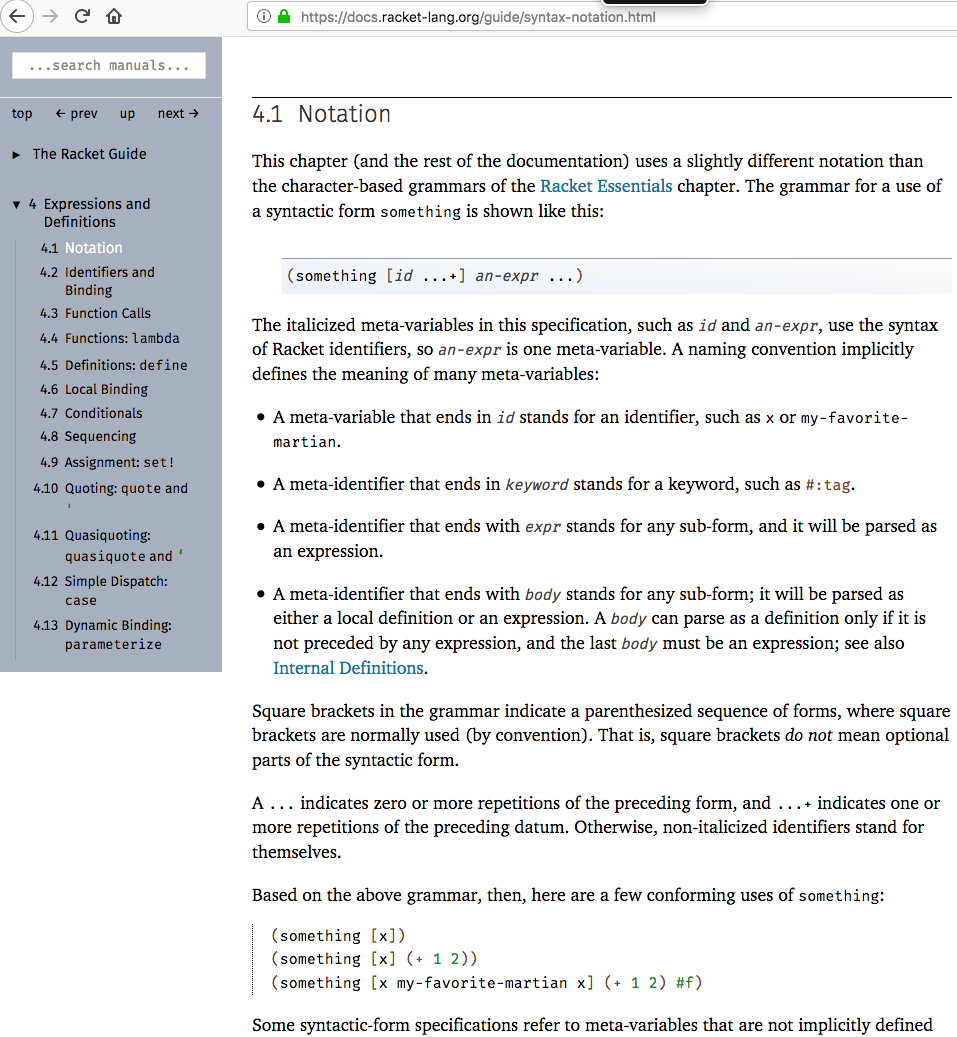# Syntactic Meaning of Variable

By Xah Lee. Date: . Last updated: .

in logic and formal language theory, there's a syntax problem. should ‹x› == ‹x› return true or false? In BNF, compiler, they can be true or false. In math, it's always true. In Mathematica symbolic pattern matching it's true (i think in haskell ocaml too)

the bottom of the issue is, does a named variable/unknown represent a SPECIFIC value? If so, ‹x›==‹x› is true. but if ‹x› represents any unknown (such as arbitrary string in a formal language), not specific, then ‹x›==‹x› can be true or false.

my interest is originally from writing docs. eg i want to say「‹x›.isPrototypeOf(‹x›)」 return false. see JS: Object.prototype.isPrototypeOf

but that depends on what the notation ‹x› is meant.

If we use the notation ‹x› to mean a specific unknown value, then, it is correct to say 「‹x›.isPrototypeOf(‹x›)」 return false, in JavaScript. Otherwise, the statement would be incorrect.

In racket lisp language's documentation, it also uses italized text for variable, extensively, and thus have the same issue.

example:Racket lang doc 4.1 notation [2018-07-26 from https://docs.racket-lang.org/guide/syntax-notation.html ]

but i haven't seen occassions of ‹x›==‹x› occur to determine what is the italized notation means exactly.

so, i wanted to think about, look at, explore, what system of formal lang or meaning of a italic var should be defined, pro and cons, etc. eg look at coq idris etc. it's not a problem i actually need to solve, but alone the way with my study of proof systems/formal lang.

to recap:

In traditional math notation, a variable ‹x› (consider ‹x› as italized x), usually means a specific value. So, mathematicians would write reflecive property as x = x. So, here, variable/symbols of the same name means the same value. (thus, in our context, ‹x›==‹x› is always true.)

in BNF system, variable is called non-terminal, and traditionally written as all caps, e.g. X. So, whether X is equals to itself, depends on what eventual terminals are used to replace X. So, X == X can mean true or false. (in our context, that means, ‹x›==‹x› can be true or false.)

In Mathematica and haskel ocaml etc pattern matching system, a named pattern has a specific value. So that means, ‹x›==‹x› is always true.

so am wondering, what's the similar situation/system if any, used in coq, idris, agda, etc to represent a variable, unknown, or meta variable (as the Racket lang doc discussed.).

If you have a question, put \$5 at patreon and message me.